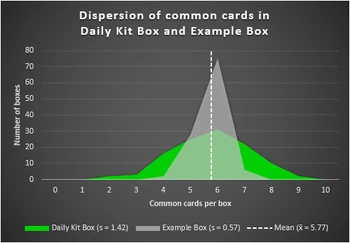## FANDOM

4,795 PagesSamples from two boxes with the same mean but different dispersion. The amount of common cards in Daily Kit Boxes (as of July 18, 2019) is more dispersed than in the (fictitious) Example Boxes, also reflected by the larger standard deviation s.

In statistics, dispersion (also called variability, scatter, or spread) is the extent to which a distribution is stretched or squeezed. Common examples of measures of statistical dispersion are the variance or the standard deviation.

## Measures

A measure of statistical dispersion is a nonnegative real number that is zero if all the data are the same and increases as the data become more diverse.

Most measures of dispersion have the same units as the quantity being measured. In other words, if the measurements are in Pro Kit cards, so is the measure of dispersion.